Search by Topic

Resources tagged with Representing similar to Birthday Sharing:

Filter by: Content type:
Age range:
Challenge level:

There are 15 resultsCatrina's Cards

Age 5 to 7 Challenge Level:

How would you find out how many football cards Catrina has collected?Birthday Sharing

Age 5 to 7 Challenge Level:

It's Sahila's birthday and she is having a party. How could you answer these questions using a picture, with things, with numbers or symbols?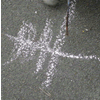Children's Mathematical Graphics: Understanding the Key Concept

Age 5 to 7

In this article for teachers, Elizabeth Carruthers and Maulfry Worthington explore the differences between 'recording mathematics' and 'representing mathematical thinking'.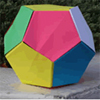Dodecamagic

Age 7 to 11 Challenge Level:

Here you see the front and back views of a dodecahedron. Each vertex has been numbered so that the numbers around each pentagonal face add up to 65. Can you find all the missing numbers?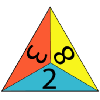One Big Triangle

Age 5 to 7 Challenge Level:

Make one big triangle so the numbers that touch on the small triangles add to 10.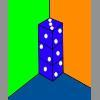Dice in a Corner

Age 7 to 11 Challenge Level:

How could you arrange at least two dice in a stack so that the total of the visible spots is 18?Going for Gold

Age 7 to 11 Challenge Level:

Looking at the 2012 Olympic Medal table, can you see how the data is organised? Could the results be presented differently to give another nation the top place?Different Sizes

Age 5 to 11 Challenge Level:

A simple visual exploration into halving and doubling.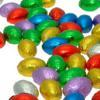Let Us Divide!

Age 7 to 11 Challenge Level:

Look at different ways of dividing things. What do they mean? How might you show them in a picture, with things, with numbers and symbols?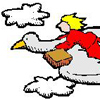A Flying Holiday

Age 7 to 11 Short Challenge Level:

Follow the journey taken by this bird and let us know for how long and in what direction it must fly to return to its starting point.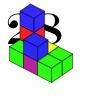28 and It's Upward and Onward

Age 7 to 11 Challenge Level:

Can you find ways of joining cubes together so that 28 faces are visible?3D Stacks

Age 7 to 14 Challenge Level:

Can you find a way of representing these arrangements of balls?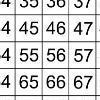That Number Square!

Age 5 to 11 Challenge Level:

Exploring the structure of a number square: how quickly can you put the number tiles in the right place on the grid?Olympic Turns

Age 7 to 11 Challenge Level:

This task looks at the different turns involved in different Olympic sports as a way of exploring the mathematics of turns and angles.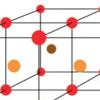Finding 3D Stacks

Age 7 to 11 Challenge Level:

Can you find a way of counting the spheres in these arrangements?